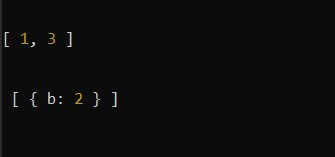GeeksforGeeks App
Open AppBrowser
Continue

# Lodash _.differenceWith() Method

The _.differenceWith() method is similar to _.difference() method that returns the array containing the values that are in the first array not in the second array but in _.differenceWith() all the elements of the first array are compared with the second array by applying comparison provided in third. It may be a little complex to understand by reading this but it will become simple when you see the example.

Syntax:

`_.differenceWith(array, [values], [comparator])`

Parameters: This method accept three parameters as mentioned above and described below:

• array: This parameter holds the array that values are checked or inspect.
• values: This parameter holds the value that need to be removed.
• comparator: This parameter holds the comparison invoked per element.

Return Value: This method returns an array according to the condition explained above.

Example 1:

 `const _ = require(``'lodash'``)`` ` `let x = [1, 2, 3]`` ` `let y = [2, 4, 5]`` ` `let result = _.differenceWith(x, y, _.isEqual);`` ` `console.log(result);`

Here, `const _ = require('lodash')` is used to import the lodash library into the file.

Output:

`[1, 3]`

So, here each element of the first array is compared with each element of the second array according to the third comparator, in our case its _.isEqual. So, if the value becomes equal it removes it.

Example 2:

 `const _ = require(``'lodash'``);`` ` `let x = [{a: 1}, {b: 2}, 6]`` ` `let y = [{a: 1}, 7, 6]`` ` `let result = _.differenceWith(x, y, _.isEqual);`` ` `console.log(result);`

Output:

```[{b: 2}]
```

Example 3:

 `const _ = require(``'lodash'``);`` ` `let x1 = [1, 2, 3]`` ` `let y1 = [2, 4, 5]`` ` `let result1 = _.differenceWith(x1, y1, _.isEqual);`` ` `console.log(result1);`` ` `let x2 = [{a: 1}, {b: 2}, 6]`` ` `let y2 = [{a: 1}, 7, 6]`` ` `let result2 = _.differenceWith(x2, y2, _.isEqual);`` ` `console.log(``'\n\n'``, result2);`

Output:Note: This will not work in normal JavaScript because it requires the library lodash to be installed.

My Personal Notes arrow_drop_up
Related Tutorials